JEE  >  35 Year JEE Previous Year Questions: Electrochemistry

# 35 Year JEE Previous Year Questions: Electrochemistry

Test Description

## 35 Questions MCQ Test Chemistry for JEE | 35 Year JEE Previous Year Questions: Electrochemistry

35 Year JEE Previous Year Questions: Electrochemistry for JEE 2023 is part of Chemistry for JEE preparation. The 35 Year JEE Previous Year Questions: Electrochemistry questions and answers have been prepared according to the JEE exam syllabus.The 35 Year JEE Previous Year Questions: Electrochemistry MCQs are made for JEE 2023 Exam. Find important definitions, questions, notes, meanings, examples, exercises, MCQs and online tests for 35 Year JEE Previous Year Questions: Electrochemistry below.
Solutions of 35 Year JEE Previous Year Questions: Electrochemistry questions in English are available as part of our Chemistry for JEE for JEE & 35 Year JEE Previous Year Questions: Electrochemistry solutions in Hindi for Chemistry for JEE course. Download more important topics, notes, lectures and mock test series for JEE Exam by signing up for free. Attempt 35 Year JEE Previous Year Questions: Electrochemistry | 35 questions in 70 minutes | Mock test for JEE preparation | Free important questions MCQ to study Chemistry for JEE for JEE Exam | Download free PDF with solutions
 1 Crore+ students have signed up on EduRev. Have you?
35 Year JEE Previous Year Questions: Electrochemistry - Question 1

### Conductivity (unit Siemen’s S) is directly proportional toarea of the vessel and the concentration of the solution in itand is inversely proportional to the length of the vesselthen the unit of the constant of proportionality is                

Detailed Solution for 35 Year JEE Previous Year Questions: Electrochemistry - Question 1

given :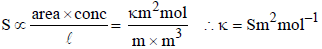35 Year JEE Previous Year Questions: Electrochemistry - Question 2

### EMF of a cell in terms of reduction potential of its left andright electrodes is        

Detailed Solution for 35 Year JEE Previous Year Questions: Electrochemistry - Question 2

Ecell = Reduction potential of cathode (right)
– Reduction potential of anode (left)
= Eright – Eleft.

35 Year JEE Previous Year Questions: Electrochemistry - Question 3

### What will be the emf for the given cell  Pt | H2 (P1) | H+ (aq) | | H2 (P2) | Pt

Detailed Solution for 35 Year JEE Previous Year Questions: Electrochemistry - Question 3

Oxidation half call:-The net cell reaction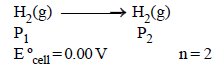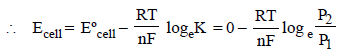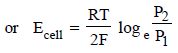35 Year JEE Previous Year Questions: Electrochemistry - Question 4

Which of the following reaction is possible at anode?

Detailed Solution for 35 Year JEE Previous Year Questions: Electrochemistry - Question 4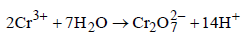O.S. of Cr changes from +3 to +6 by loss of electrons. At anode oxidation takes place.

35 Year JEE Previous Year Questions: Electrochemistry - Question 5

When the sample of copper with zinc impurity is to be purified by electrolysis, the appropriate electrodes are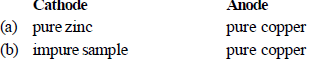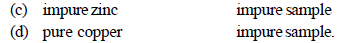Detailed Solution for 35 Year JEE Previous Year Questions: Electrochemistry - Question 5

Pure metal always deposits at cathode

35 Year JEE Previous Year Questions: Electrochemistry - Question 6

Which of the following is a redox reaction? 

Detailed Solution for 35 Year JEE Previous Year Questions: Electrochemistry - Question 6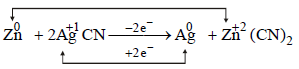35 Year JEE Previous Year Questions: Electrochemistry - Question 7

For a cell reaction involving a two-electron change, thestandard e.m.f. of the cell is found to be 0.295 V at 25ºC. The equilibrium constant of the reaction at 25ºC will be               [2003

Detailed Solution for 35 Year JEE Previous Year Questions: Electrochemistry - Question 7

The equilibrium constant is related to the standard emf of cell by the expression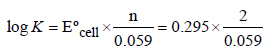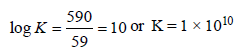35 Year JEE Previous Year Questions: Electrochemistry - Question 8

Standard reduction electrode potentials of three metals A, B& C are respectively + 0.5 V, – 3.0 V & –1.2 V. The reducingpowers of these metals are 

Detailed Solution for 35 Year JEE Previous Year Questions: Electrochemistry - Question 8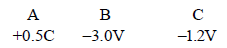NOTE : The higher the negative value of reduction potential, the more is the reducing power.
Hence B > C > A.

35 Year JEE Previous Year Questions: Electrochemistry - Question 9

When during electrolysis of a solution of AgNO3 9650coulombs of charge pass through the electroplating bath,the mass of silver deposited on the cathode will be

Detailed Solution for 35 Year JEE Previous Year Questions: Electrochemistry - Question 9

When 96500 coulomb of electricity is passed through the electroplating bath the amount of Ag deposited = 108g
∴ when 9650 coulomb of electricity is passed deposited Ag.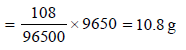35 Year JEE Previous Year Questions: Electrochemistry - Question 10

For the redox reaction :
Zn(s) +Cu2+ (0.1M)→ Zn2+ (1M) + Cu(s) taking place in a cell,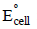is 1.10 volt. Ecell for the cell will  be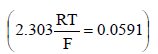Detailed Solution for 35 Year JEE Previous Year Questions: Electrochemistry - Question 10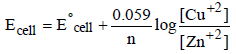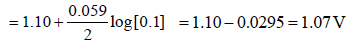35 Year JEE Previous Year Questions: Electrochemistry - Question 11

Several blocks of magnesium are fixed to the bottom of a ship to                   

Detailed Solution for 35 Year JEE Previous Year Questions: Electrochemistry - Question 11

Magnesium, on account of its lightness, great affinity for oxygen and toughness is used in ship. Being a lighter element, magnesium makes the ship lighter when it is fixed to the bottom of the ship.

35 Year JEE Previous Year Questions: Electrochemistry - Question 12

In a hydrogen-oxygen fuel cell, combustion of hydrogenoccurs to               

Detailed Solution for 35 Year JEE Previous Year Questions: Electrochemistry - Question 12

In H2 -O2 fuel cell, the combustion of H2 occurs to create potential difference between the two electrodes

35 Year JEE Previous Year Questions: Electrochemistry - Question 13

Consider the following Eº values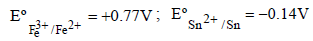Under standard conditions the potential for the reaction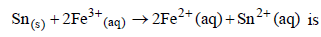Detailed Solution for 35 Year JEE Previous Year Questions: Electrochemistry - Question 13

Fe3+ + e- → Fe2+ΔG° = -1x Fx0.77
Sn2+ + 2e- → Sn(s)ΔG° = -2 x F(-0.14)
for Sn(s) + 2Fe3+ (aq) → 2Fe2+ (aq) +Sn2+ (aq)
∴ Standard potential for the given reaction

∴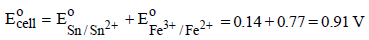35 Year JEE Previous Year Questions: Electrochemistry - Question 14

The standard e.m.f. of a cell involving one electron changeis found to be 0.591 V at 25ºC. The equilibrium constant ofthe reaction is (F = 96,500 C mol–1; R = 8.314 JK–1 mol–1)                             

Detailed Solution for 35 Year JEE Previous Year Questions: Electrochemistry - Question 14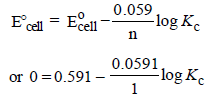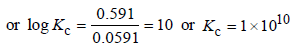35 Year JEE Previous Year Questions: Electrochemistry - Question 15

The limiting molar conductivities Lº for NaCl, KBr and KClare 126, 152 and 150 S cm2 mol–1 respectively. The Lº forNaBr is 

Detailed Solution for 35 Year JEE Previous Year Questions: Electrochemistry - Question 15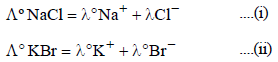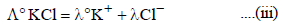operating (i) + (ii) - (iii)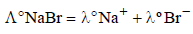= 126 +152 -150 = 128 S cm2 mol-1

35 Year JEE Previous Year Questions: Electrochemistry - Question 16

In a cell that utilises the reaction  Zn(s) + 2H+ (aq) → Zn2++ H2(g) addition of H2SOto cathode compartment, will          

Detailed Solution for 35 Year JEE Previous Year Questions: Electrochemistry - Question 16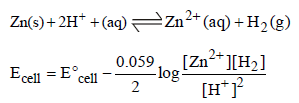35 Year JEE Previous Year Questions: Electrochemistry - Question 17

The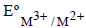values for Cr, Mn, Fe and Co are – 0.41, + 1.57, + 0.77 and + 1.97V respectively. For which one of these
metals the change in oxidation state from +2 to +3 is easiest?               

Detailed Solution for 35 Year JEE Previous Year Questions: Electrochemistry - Question 17

The given values show that Cr has maximum oxidation potental, therefore its oxidation will be easiest. (Change the sign to get the oxidation values)

35 Year JEE Previous Year Questions: Electrochemistry - Question 18

For a spontaneous reaction the DG, equilibrium constant (K) andwill be respectively 

Detailed Solution for 35 Year JEE Previous Year Questions: Electrochemistry - Question 18

NOTE : For spontaneous reaction DG should be negative. Equilibrium constant should be more than one

(ΔG = – 2.303 RT log Kc, If Kc = 1 then ΔG = 0; If Kc < 1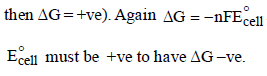35 Year JEE Previous Year Questions: Electrochemistry - Question 19

The highest electrical conductivity of the following aqueoussolutions is of                     

Detailed Solution for 35 Year JEE Previous Year Questions: Electrochemistry - Question 19

Thus difluoro acetic acid being strongest acid will furnish maximum number of ions showing highest electrical conductivity. The decreasing acidic strength of the carboxylic acids given is difluoro acetic acid > fluoro acetic acid > chloro acitic acid > acetic acid.

35 Year JEE Previous Year Questions: Electrochemistry - Question 20

Aluminium oxide may be electrolysed at 1000°C to furnishaluminium metal (At. Mass = 27 amu; 1 Faraday = 96,500Coulombs). The cathode reaction is– Al3+ + 3e- → Al° To prepare 5.12 kg of aluminium metal by this method werequire

Detailed Solution for 35 Year JEE Previous Year Questions: Electrochemistry - Question 20

1 mole of e– = 1F = 96500 C
27g of Al is deposited by 3 × 96500 C
5120 g of Al will be deposited by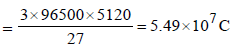35 Year JEE Previous Year Questions: Electrochemistry - Question 21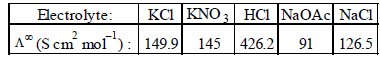Calculateusing appropriate molar conductances of the electrolytes listed above at infinite dilution in H2O
at 25°C

Detailed Solution for 35 Year JEE Previous Year Questions: Electrochemistry - Question 21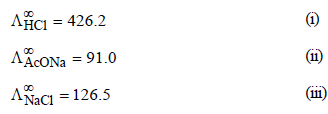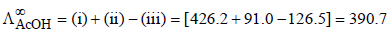35 Year JEE Previous Year Questions: Electrochemistry - Question 22

Which of the following chemical reactions depict the oxidizing beahviour of H2SO4?

Detailed Solution for 35 Year JEE Previous Year Questions: Electrochemistry - Question 22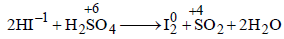reaction oxidation number of S is decreasing from + 6 to +4 hence undergoing reduction and for HI oxidation

Number of I is increasing from –1 to 0 hence
underegoing oxidation therefore H2SO4 is acting as oxidising agent.

35 Year JEE Previous Year Questions: Electrochemistry - Question 23

The molar conductivities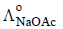and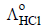at infinite dilution in water at 25ºC are 91.0 and 426.2 S cm2/mol
respectively. To calculate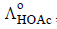, the additional value required is

Detailed Solution for 35 Year JEE Previous Year Questions: Electrochemistry - Question 23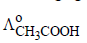is given by the following equation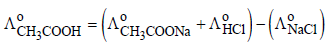Hence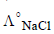is required.

35 Year JEE Previous Year Questions: Electrochemistry - Question 24

Resistance of a conductivity cell filled with a solution of an electrolyte of concentration 0.1 M is 100 W. The conductivity of this solution is 1.29 S m–1. Resistance of the same cell when filled with 0.2 M of the same solution is 520 W. The molar conductivity of 0.2 M solution of electrolyte will be                            

Detailed Solution for 35 Year JEE Previous Year Questions: Electrochemistry - Question 24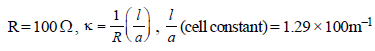Given, R = 520Ω, C = 0.2 M, μ (molar conductivity) = ?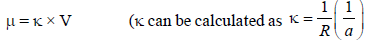now cell constant is known.)

Hence,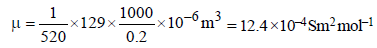35 Year JEE Previous Year Questions: Electrochemistry - Question 25

The equivalent conductances of two strong electrolytes at infinite dilution in H2O (where ions move freely through a
solution) at 25°C are given below :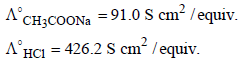What additional information/ quantity one needs to calculate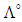of an aqueous solution of acetic acid?

Detailed Solution for 35 Year JEE Previous Year Questions: Electrochemistry - Question 25

NOTE : According to Kohlrausch’s law, molar
conductivity of weak electrolyte acetic acid (CH3COOH) can be calculated as follows: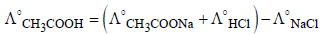∴ Value ofshould also be known for
calculating value of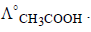35 Year JEE Previous Year Questions: Electrochemistry - Question 26

The cell, Zn | Zn2+ (1 M) || Cu2+ (1 M) | Cu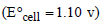was allowed to be completely discharged at 298 K. The relative
concentration of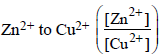is         

Detailed Solution for 35 Year JEE Previous Year Questions: Electrochemistry - Question 26

Ecell = 0; when cell is completely discharged.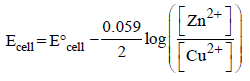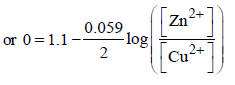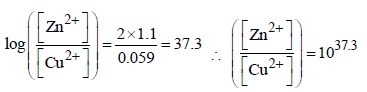35 Year JEE Previous Year Questions: Electrochemistry - Question 27

Given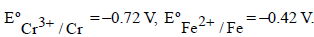The potential for the cell Cr|Cr3+ (0.1M)|| Fe2+ (0.01 M)| Fe is         

Detailed Solution for 35 Year JEE Previous Year Questions: Electrochemistry - Question 27

From the given representation of the cell, Ecell can be found as follows.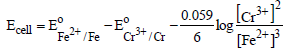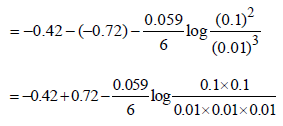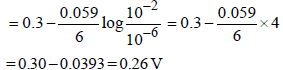Hence option (d) is correct answer.

35 Year JEE Previous Year Questions: Electrochemistry - Question 28

In a fuel cell methanol is used as fuel and oxygen gas is used as an oxidizer. The reaction is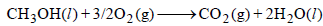At 298 K standard Gibb’s energies of formation for CH3OH(l), H2O(l) and and CO2 (g) are –166.2,  –237.2 and –394.4 kJ
mol–1 respectively. If standard enthalpy of combustion of methonal is – 726 kJ mol–1, efficiency of the fuel cell will be:



Detailed Solution for 35 Year JEE Previous Year Questions: Electrochemistry - Question 28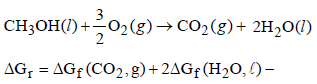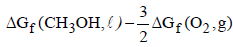= – 394.4 + 2 (–237.2) – (–166.2) – 0
= – 394.4 – 474.4 + 166.2 = – 702.6 k J
% efficiency =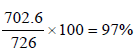35 Year JEE Previous Year Questions: Electrochemistry - Question 29

Given: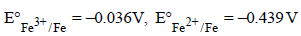The value of standard electrode potential for the change, Fe3+ (aq) + e →Fe2+ (aq) will be:



Detailed Solution for 35 Year JEE Previous Year Questions: Electrochemistry - Question 29

Given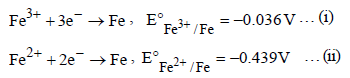we have to calculate

Fe3+ + e → Fe2+ … (iii)
As we know that ΔG = –nFE
Thus for reaction (iii)

ΔG = ΔG1 - ΔG ; – nFE° = – nFE1 – (–nFE2)
–nFE° = nFE2 – nFE1
–1FE° = 2× 0.439F – 3 × 0.036 F
–1 FE° = 0.770 F ∴ E° = – 0.770V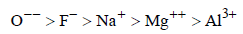35 Year JEE Previous Year Questions: Electrochemistry - Question 30

The Gibbs energy for the decomposition of Al2O3 at 500°C is as follows :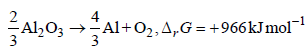The potential difference needed for electrolytic reductionof Al2O3 at 500°C is at least                      

Detailed Solution for 35 Year JEE Previous Year Questions: Electrochemistry - Question 30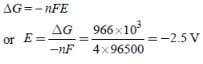35 Year JEE Previous Year Questions: Electrochemistry - Question 31

The correct order of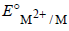values with negative sign for the four successive elements Cr, Mn, Fe and Co is 

Detailed Solution for 35 Year JEE Previous Year Questions: Electrochemistry - Question 31

The value of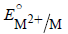for given metal ions are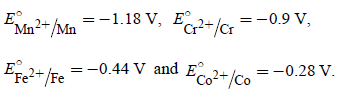The correct order ofvalues without
considering negative sign would be

Mn2+ > Cr2+ > Fe2+ > Co2+.

35 Year JEE Previous Year Questions: Electrochemistry - Question 32

The reduction potential of hydrogen half-cell will be negative if :



Detailed Solution for 35 Year JEE Previous Year Questions: Electrochemistry - Question 32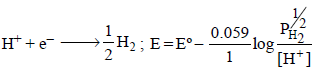Now if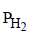=  2 atm and [H+] = 1M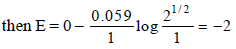35 Year JEE Previous Year Questions: Electrochemistry - Question 33

The standard reduction potentials for Zn2+/Zn, Ni2+/Ni and Fe2+/Fe are –0.76,–0.23 and –0.44 V respectively.
The reaction X+Y2+ →X2++Y will be spontaneous when :

Detailed Solution for 35 Year JEE Previous Year Questions: Electrochemistry - Question 33

For a spontaneous reaction DG must be –ve
Since ΔG = – nFE°
Hence for ΔG to be -ve ΔE° has to be positive. Which is possible when X = Zn, Y = Ni
Zn + Ni++ →  Zn++ + Ni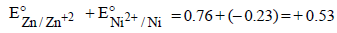35 Year JEE Previous Year Questions: Electrochemistry - Question 34

Given :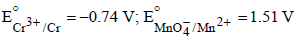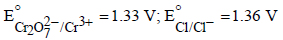Based on the data given above, strongest oxidising agentwill be : [JEE M 2013]

Detailed Solution for 35 Year JEE Previous Year Questions: Electrochemistry - Question 34

higher the value of standard reduction potential
stronger will be the oxidising agent, hence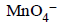is  the strongest oxidising agent.

35 Year JEE Previous Year Questions: Electrochemistry - Question 35

Resistance of 0.2 M solution of an electrolyte is 50 Ω.The specific conductance of the solution is 1.4 S m–1.The resistance of 0.5 M solution of the same electrolyte is 280 Ω. The molar conductivity of 0.5 M solution of the electrolyte in S m2 mol–1 is:

[JEE M 2014]

Detailed Solution for 35 Year JEE Previous Year Questions: Electrochemistry - Question 35

Given for 0.2 M solution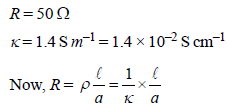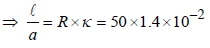For 0.5 M solution
R = 280 Ω; k = ?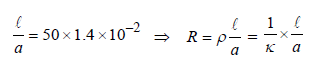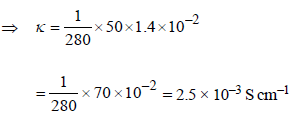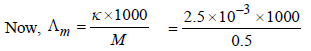= 5 S cm2 mol–1 = 5 × 10–4 S m2 mol–1

## Chemistry for JEE

322 videos|586 docs|368 tests
 Use Code STAYHOME200 and get INR 200 additional OFF Use Coupon Code
Information about 35 Year JEE Previous Year Questions: Electrochemistry Page
In this test you can find the Exam questions for 35 Year JEE Previous Year Questions: Electrochemistry solved & explained in the simplest way possible. Besides giving Questions and answers for 35 Year JEE Previous Year Questions: Electrochemistry, EduRev gives you an ample number of Online tests for practice

## Chemistry for JEE

322 videos|586 docs|368 tests